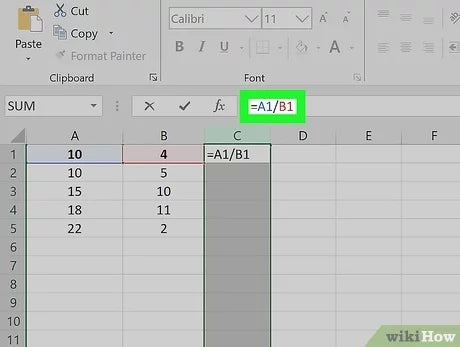Blog

# How to Divide Two Cells in Excel?

If you’re looking for a quick and easy way to divide two cells in Excel, then you’ve come to the right place! In this article, we’ll provide you with a step-by-step guide on how to divide two cells in Excel. We’ll explain how to do this with both the simple formula and the array formula, so no matter your level of expertise, you’ll be able to complete the task with ease. So, let’s get started and see how to divide two cells in Excel!## How to Divide Two Cells in Excel

Dividing two cells in Excel is a simple task that can be accomplished within a few steps. Whether you need to calculate a value for a single data set or multiple sets, Excel can easily divide the two cells for you. Here’s how to do it.

### Step 1: Select the Cell

The first step to dividing two cells in Excel is to select the cell which you’d like to use for the calculation. To do this, simply click on the cell with your mouse and the cell will be highlighted.

### Step 2: Enter the Formula

After selecting the cell, the next step is to enter the formula for the calculation. To divide two cells, you’ll need to use the “/” sign. For example, if you wanted to divide cell A2 by cell B2, you’d enter the following formula: “=A2/B2”.

### Step 3: Calculate the Result

Once you’ve entered the formula, the next step is to calculate the result. To do this, simply press the “Enter” key on your keyboard and the result of the calculation will be displayed in the cell.

## How to Divide Multiple Cells in Excel

Dividing multiple cells in Excel is a bit more complicated than dividing two cells, but it can still be done with a few simple steps. Here’s how to do it.

### Step 1: Select the Cells

The first step to dividing multiple cells in Excel is to select the cells which you’d like to use for the calculation. To do this, simply click and drag your mouse over the cells you’d like to include in the calculation.

### Step 2: Enter the Formula

After selecting the cells, the next step is to enter the formula for the calculation. To divide multiple cells, you’ll need to use the “/” sign and the “SUM” function. For example, if you wanted to divide cell A2 by cells B2 to B5, you’d enter the following formula: “=A2/SUM(B2:B5)”.

### Step 3: Calculate the Result

Once you’ve entered the formula, the next step is to calculate the result. To do this, simply press the “Enter” key on your keyboard and the result of the calculation will be displayed in the cell.

### What is a Cell in Excel?

A cell in Excel is a single rectangular box in a worksheet. Cells contain data, such as numerical values, text, or formulas. Each cell has a unique address that can be used to reference it. Cells can be combined to form larger units, such as a range, a table, or a pivot table. Cells are the building blocks of a worksheet and can be manipulated in various ways.

### How Do I Divide Two Cells in Excel?

Division in Excel can be done by using the division operator (/) to calculate the result of dividing two cells. First, select the cell that contains the formula that you want to divide in two cells. Then type the division operator followed by the cell reference of the cell that you want to divide by. Finally, press Enter to get the result. You can also use the cell references in a formula to divide two cells. For example, =A1/A2 will divide the contents of cell A1 by the contents of cell A2.

### What is the Syntax for Dividing Cells in Excel?

The syntax for dividing two cells in Excel is to type the division operator (/) followed by the cell reference of the cell that you want to divide by. For example, =A1/A2 will divide the contents of cell A1 by the contents of cell A2. You can also use the cell references in a formula to divide two cells.

### What are the Benefits of Dividing Cells in Excel?

Dividing cells in Excel can be beneficial for a variety of reasons. Dividing cells can help you quickly compare the results of two different formulas or calculations. This is especially useful when you are trying to determine the percentage of one number in relation to another. Dividing cells can also help you quickly identify trends or changes in datasets.

### Are There Any Tips for Dividing Cells in Excel?

Yes, there are several tips that you should keep in mind when dividing cells in Excel. First, make sure that you are using the correct syntax in your formulas. Also, be sure to double-check your results to ensure accuracy. Finally, you can use cell references to quickly select multiple cells at once and apply the same formula to all of them.

### What are Some Common Errors When Dividing Cells in Excel?

Some of the most common errors when dividing cells in Excel include using an incorrect formula syntax or referencing the wrong cell. Another common error is dividing by zero, which will result in an error message. Additionally, if the cells that you are attempting to divide contain text or blank cells, this will also result in an error.

Implementing the steps in this article, you can easily divide two cells in Excel and make your data calculations even easier. No matter how complex your data is, Excel can help you find the right answers. With its powerful features, you can use formulas and pivot tables to make the most out of your data. Keep in mind that Excel is a powerful tool, and with the right knowledge, you can make it work for you.